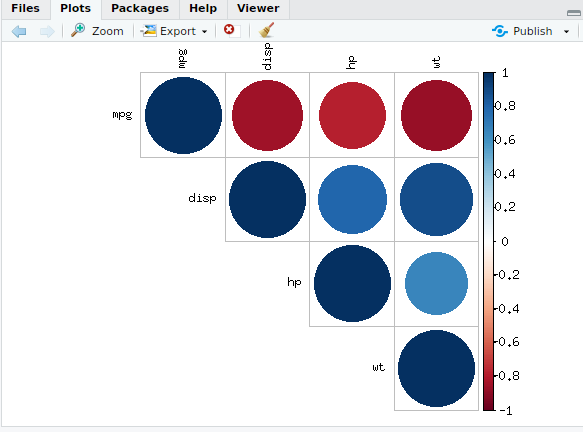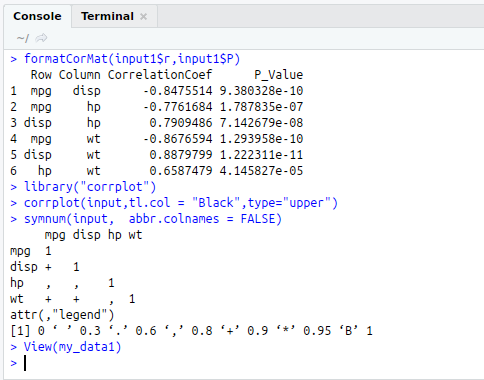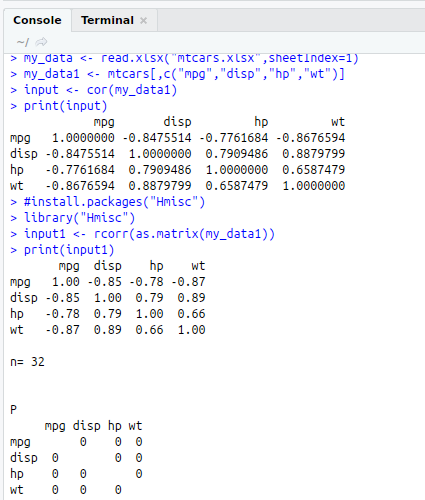• #5, First Floor, 4th Street Dr. Subbarayan Nagar Kodambakkam, Chennai-600 024 Landmark : Samiyar Madam
• pro@slogix.in
• +91- 81240 01111

### How to compute Correlation Matrix and Visualize it in R?

###### Description

To compute the correlation matrix and visualize it using R programming.

#### Correlation:

A correlation is a single number that describes the degree of relationship between two variables.

#### Correlation Matrix:

A correlation matrix is a table showing correlationcoefficients between sets of variables

R Function : cor(Data Frame)

#### Correlation Matrix with significance levels:

R Package : Hmisc

R Function :rcorr(Correlation Matrix)

#### Methods for Visualizing Correlation Matrix:

corrplot()function to plot Correlogram

symnum()function

Scatter plot

###### Sapmle Code

#Correlation and Correlation Matrix

#Get and Set Working Directory
print(getwd())
setwd(“/home/soft13”)
getwd()

#install.packages(“xlsx”)
library(“xlsx”)
my_data1<-mtcars[,c(“mpg”,”disp”,”hp”,”wt”)]
View(my_data1)

#Compute Correlation Matrix
input<-cor(my_data1)
print(input)

#Correlation Matrix with significance levels
#install.packages(“Hmisc”)
library(“Hmisc”)
input1<-rcorr(as.matrix(my_data1))
print(input1)

#Extracting Correlation Coefficients and P- Values
round(input1\$r,2)
input1\$P

#Formatting Correlation Matrix

formatCorMat<-function(corr,pval){
upper<-upper.tri(corr)
data.frame(
Row = rownames(corr)[row(corr)[upper]],
Column = colnames(corr)[col(corr)[upper]],
CorrelationCoef = corr[upper],
P_Value = pval[upper],
stringsAsFactors = FALSE
)
}
formatCorMat(input1\$r,input1\$P)

#Visualizing Correlation Matrix
#Corrplot()
library(“corrplot”)
corrplot(input,tl.col = “Black”,type=”upper”)
#symnum()
symnum(input, abbr.colnames = FALSE)

###### Screenshots